# Category: stories

Fortunately, there are a few certainties left in life:

In spring, you might expect the next instalment of Connes’ and Consani’s quest for Gabriel’s topos. Here’s the latest: $\overline{\mathbf{Spec}(\mathbb{Z})}$ and the Gromov norm.

Every half year or so, Mochizuki’s circle-of-friends tries to create some buzz announcing the next IUTeich-workshop. I’ll spare you the link, if you are still interested, follow math_jin or IUTT_bot_math_jin on Twitter.

And then, there’s the never-ending story of Grothendieck’s griboullis, kept alive by the French journalist and author Philippe Douroux.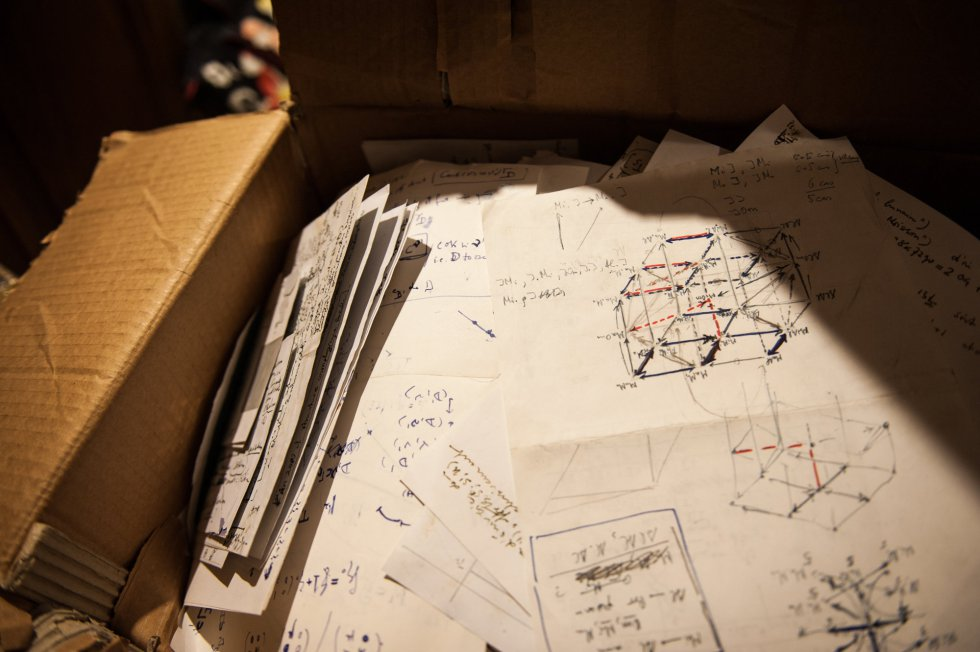Alexandre Grothendieck : une mathématique en cathédrale gothique, an article (in French) by Philippe Douroux in Le Monde, May 6th (behind paywall).

L’histoire étonnante des archives du mathématicien Alexandre Grothendieck, an article (in French) on France Inter by Mathieu Vidar, based on info from Philippe Douroux.

Les archives mystérieuses de Alexandre Grothendieck, a podcast of a broadcast on France Inter on June 10th. Interesting interview (in French) with Philippe Douroux and the French mathematician Etienne Ghys (with a guest appearance by Luc Illusie).

El enigmático legado de un genio de las matemáticas, an article (in Spanish) in El Pais, May 13th, with 8 photos of some of the Gribouillis. The two pictures in this post are taken from this article.

So, what’s the latest on the 70.000+ pages left by Grothendieck?

As far as i know, the Mormoiron part of the gribouillis is still at the University of Montpellier, and has been made available online at the Grothendieck archives.

The Lasserre part of the gribouillis is still in a cellar in Paris’ Saint-Germain-des-Prés, belonging to Jean-Bernard Gillot. The French national library cannot take possession of the notes before a financial agreement is reached with Grothendieck’s children (French law does not allow children to be disinherited).

And there’s a dispute about the price to be paid. The notes were estimated at 45.000 Euros, but some prefer to believe that they may be worth several millions of dollars.

It all depends on their mathematical content.

Unfortunately, pictures claimed to be of the Lasserre notes (such as the one above) are in fact from the Mormoiron/Montpellier notes, which do indeed contain interesting mathematics.

But, it is very unlikely that the Lasserre notes contain (math) surprises. Probably, most of them look like this one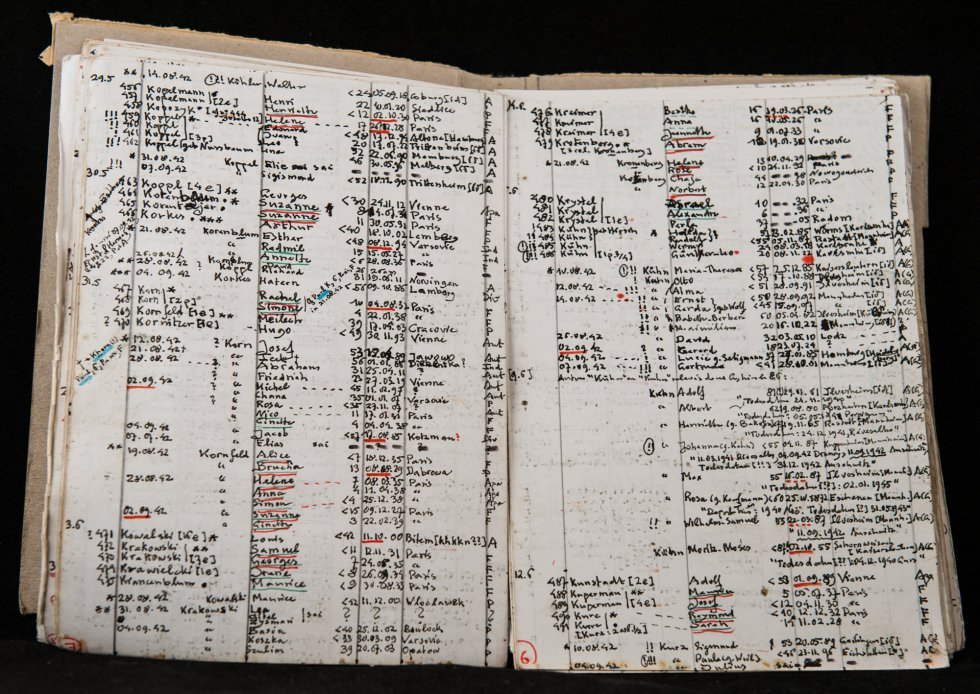endless lists of people deported by the Nazis to extermination camps in WW2.

Or, as Philippe Douroux is quoted in the El Pais piece: “I think it’s a treasure, maybe not a mathematical one, but a human one. It’s a descent into the hell of one the best organised brains in the world.”The film made by Catherine Aira and Yves Le Pestipon “Alexandre Grothendieck: On the Paths of a Genius” (on the quest for G’s last hideout in the French Pyrenees) can now be watched on YouTube (with English subtitles)

Yesterday, there was an interesting post by John Baez at the n-category cafe: The Riemann Hypothesis Says 5040 is the Last.

The 5040 in the title refers to the largest known counterexample to a bound for the sum-of-divisors function
$\sigma(n) = \sum_{d | n} d = n \sum_{d | n} \frac{1}{n}$

In 1983, the french mathematician Guy Robin proved that the Riemann hypothesis is equivalent to
$\frac{\sigma(n)}{n~log(log(n))} < e^{\gamma} = 1.78107...$ when $n > 5040$.

The other known counterexamples to this bound are the numbers 3,4,5,6,8,9,10,12,16,18,20,24,30,36,48,60,72,84,120,180,240,360,720,840,2520.In Baez’ post there is a nice graph of this function made by Nicolas Tessore, with 5040 indicated with a grey line towards the right and the other counterexamples jumping over the bound 1.78107…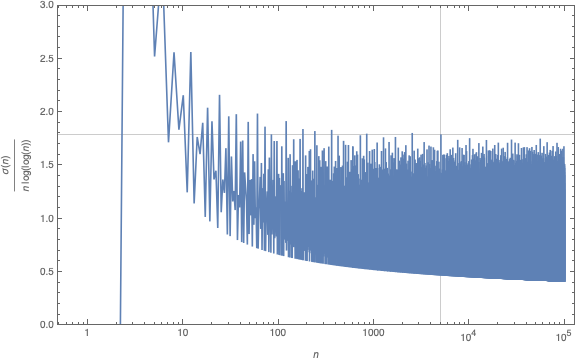Robin’s theorem has a remarkable history, starting in 1915 with good old Ramanujan writing a part of this thesis on “highly composite numbers” (numbers divisible by high powers of primes).

His PhD. adviser Hardy liked his result but called them “in the backwaters of mathematics” and most of it was not published at the time of Ramanujan’s degree ceremony in 1916, due to paper shortage in WW1.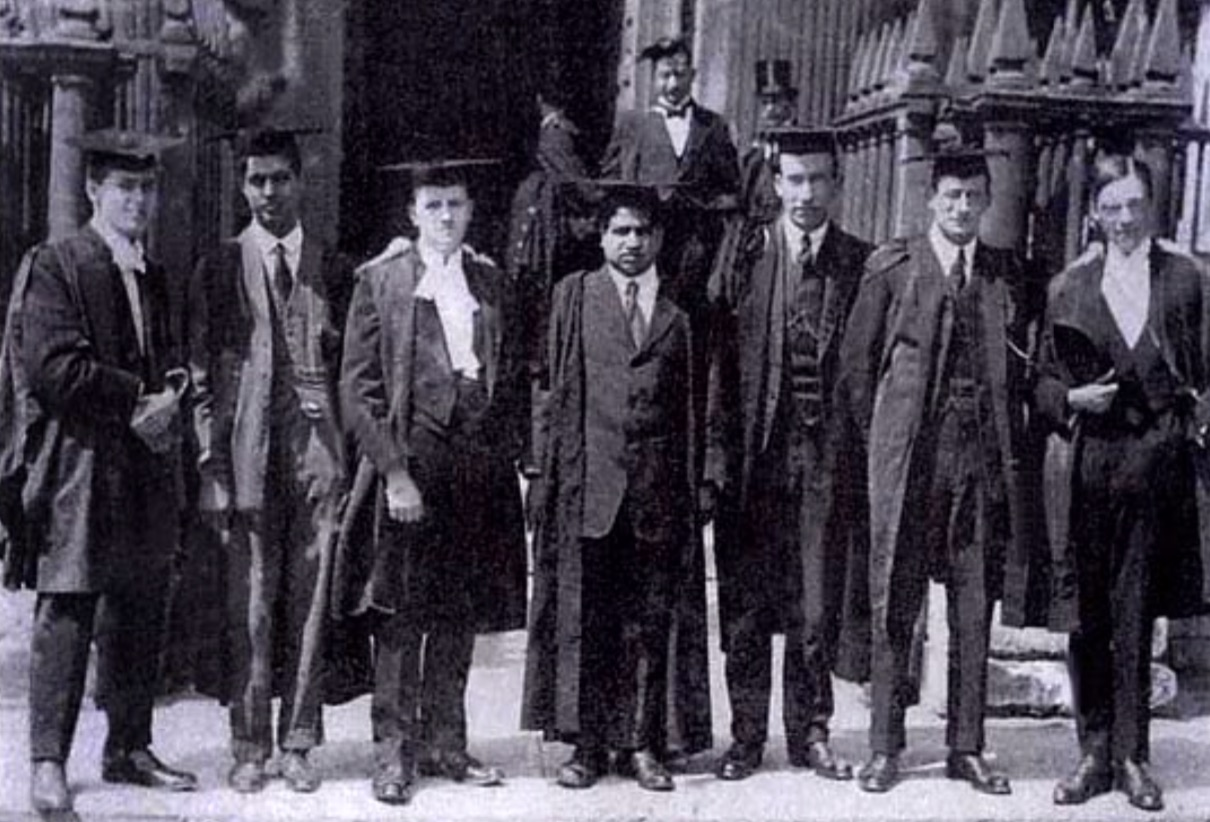When Ramanujan’s paper “Highly Composite Numbers” was first published in 1988 in ‘The lost notebook and other unpublished papers’ it became clear that Ramanujan had already part of Robin’s theorem.

Ramanujan states that if the Riemann hypothesis is true, then for $n_0$ large enough we must have for all $n > n_0$ that
$\frac{\sigma(n)}{n~log(log(n))} < e^{\gamma} = 1.78107...$ When Jean-Louis Nicolas, Robin's PhD. adviser, read Ramanujan's lost notes he noticed that there was a sign error in Ramanujan's formula which prevented him from seeing Robin's theorem.

Nicolas: “Soon after discovering the hidden part, I read it and saw the difference between Ramanujan’s result and Robin’s one. Of course, I would have bet that the error was in Robin’s paper, but after recalculating it several times and asking Robin to check, it turned out that there was an error of sign in what Ramanujan had written.”

If you are interested in the full story, read the paper by Jean-Louis Nicolas and Jonathan Sondow: Ramanujan, Robin, Highly Composite Numbers, and the Riemann Hypothesis.

What’s the latest on Robin’s inequality? An arXiv-search for Robin’s inequality shows a flurry of activity.

For starters, it has been verified for all numbers smaller that $10^{10^{13}}$…

It has been verified, unconditionally, for certain classes of numbers:

• all odd integers $> 9$
• all numbers not divisible by a 25-th power of a prime

Rings a bell? Here’s another hint:

According to Xiaolong Wu in A better method than t-free for Robin’s hypothesis one can replace the condition of ‘not divisible by an N-th power of a prime’ by ‘not divisible by an N-th power of 2’.

Further, he claims to have an (as yet unpublished) argument that Robin’s inequality holds for all numbers not divisible by $2^{42}$.

So, where should we look for counterexamples to the Riemann hypothesis?

What about the orders of huge simple groups?

The order of the Monster group is too small to be a counterexample (yet, it is divisible by $2^{46}$).

In April my Google+ account will disappear. Here I collect some G+ posts, in chronological order, having a common theme. Today, math-history (jokes and puns included).

September 20th, 2011

Was looking up pictures of mathematicians from the past and couldn’t help thinking ‘Hey, I’ve seen this face before…’

Leopold Kronecker = DSK (2/7/2019 : DSK = Dominique Strauss-Kahn)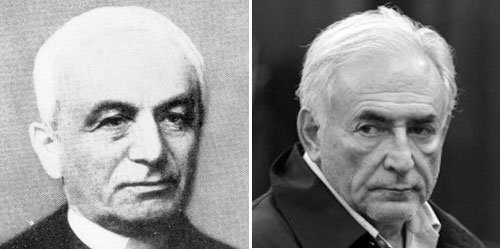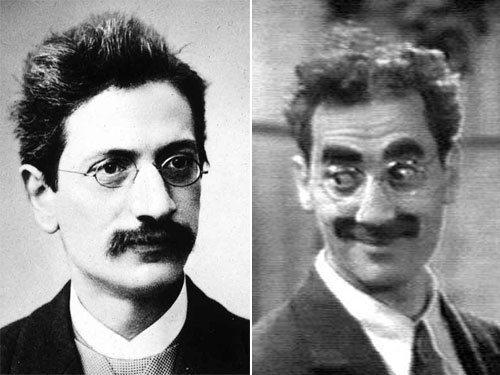June 2nd, 2012

The ‘Noether boys’

(Noether-Knaben in German) were the group of (then) young algebra students around Emmy Noether in the early 1930’s. Actually two of them were girls (Grete Hermann and Olga Taussky).

The picture is taken from a talk Peter Roquette gave in Heidelberg. Slides of this talk are now available from his website.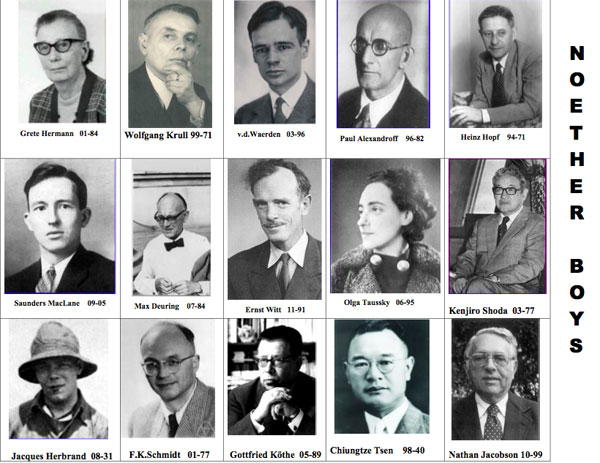In 1931 Jacques Herbrand (one of the ‘Noether boys’) fell to his death while mountain-climbing in the Massif des Écrins (France). He was just 23, but already considered one of the greatest minds of his generation.

He introduced the notion of recursive functions while proving “On the consistency of arithmetic”. In several texts on Herbrand one finds this intriguing quote by Chevalley (one of the first generation Bourbakis):

“Jacques Herbrand would have hated Bourbaki” said French mathematician Claude Chevalley quoted in Michèle Chouchan “Nicolas Bourbaki Faits et légendes” Edition du choix, 1995. («Jacques Herbrand aurait détesté Bourbaki» in the original French version).

Can anyone tell me the underlying story?

June 26th, 2012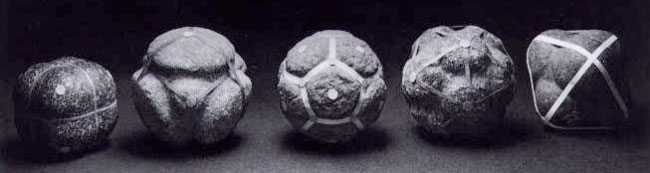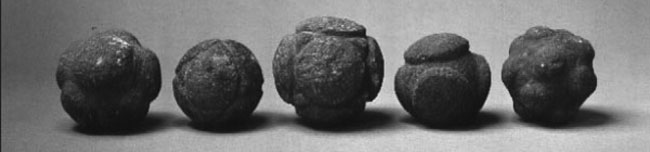I’d better point them to the latest on this then.

The return of the Scottish solids

December 19th, 2012

Mumford’s treasure map

+Pieter Belmans (re)discovered a proto-drawing of Mumford’s iconic map of Spec(Z[x]) in his ‘red book’.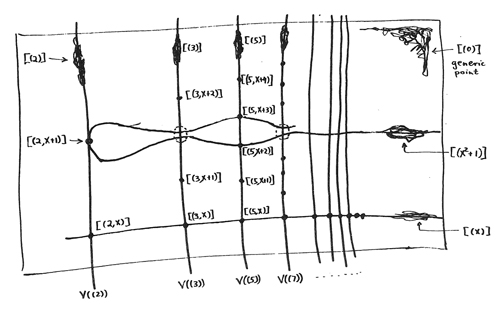The proto-pic is taken from Mumford’s ‘Lectures on curves on an algebraic surface’ p.28 and tries to depict the integral projective line. The set-up is rather classical (focussing on points of different codimension) whereas the red-book picture is more daring and has been an inspiration for generations of arithmetical geometers.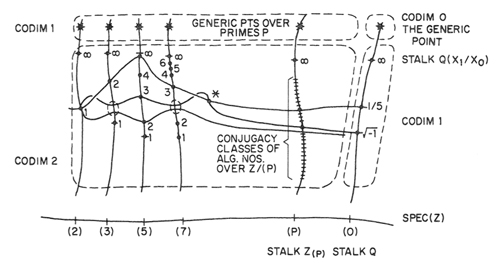Still there’s the issue of dating these maps.

Mumford himself dates the P^1 drawing 1964 (although the publication date is 66) and the red-book as 1967.

Though I’d love to hear more precise dates, I’m convinced they are about right. In the ‘Curves’-book’s preface Mumford apologises to ‘any reader who, hoping that he would find here in these 60 odd pages an easy and concise introduction to schemes, instead becomes hopelessly lost in a maze of unproven assertions and undeveloped suggestions.’ and he stresses by underlining ‘From lecture 12 on, we have proven everything that we need’.

So, clearly the RedBook was written later, and as he has written in-between his master-piece GIT i’d say Mumford’s own dating is about right.

Still, it is not a completely vacuous dispute as the ‘Curves’ book (supposedly from 1964 or earlier) contains a marvelous appendix by George Bergman on the Witt ring which would predate Cartier’s account…

Thanks to +James Borger i know of George’s take on this

“I was a graduate student taking the course Mumford gave on curves and surfaces; but algebraic geometry was not my main field, and soon into the course I was completely lost.  Then Mumford started a self-contained topic that he was going to weave in — ring schemes — and it made clear and beautiful sense to me; and when he constructed the Witt vector ring scheme, I thought about it, saw a nicer way to do it, talked with him about it and with his permission presented it to the class, and eventually wrote it up as a chapter in his course notes.
I think that my main substantive contribution was the tying together of the various prime-specific ring schemes into one big ring scheme that works for all primes.  The development in terms of power series may or may not have originated with me; I just don’t remember.”

which sounds very Bergmannian to me.

Anyway I’d love to know more about the dating of the ‘Curves’ book and (even more) the first year Mumford delivered his Red-Book-Lectures (my guess 1965-66). Thanks.
Pieter maintains an “Atlas of this picture” here

June 17th, 2013

the birthday of schemes : november 5th 1956

The wikipedia-entry linking Andre Martineau to the origin of the scheme-concept appears to rely on footnote 29 of Cartier’s ‘A mad day’s work, from Grothendieck to Connes and Kontsevich’ which reads:

“Serre first considered the set of maximal ideals of a commutative ring A subject to certain restrictions. Martineau then remarked to him that his arguments remained valid for any commutative ring, provided one takes all prime ideals instead of only maximal ideals. I then proposed a definition of schemes equivalent to the definition of Grothendieck. In my dissertation I confined myself to a framework similar to that of Chevalley, so as to avoid an excessively long exposition of the preliminaries!”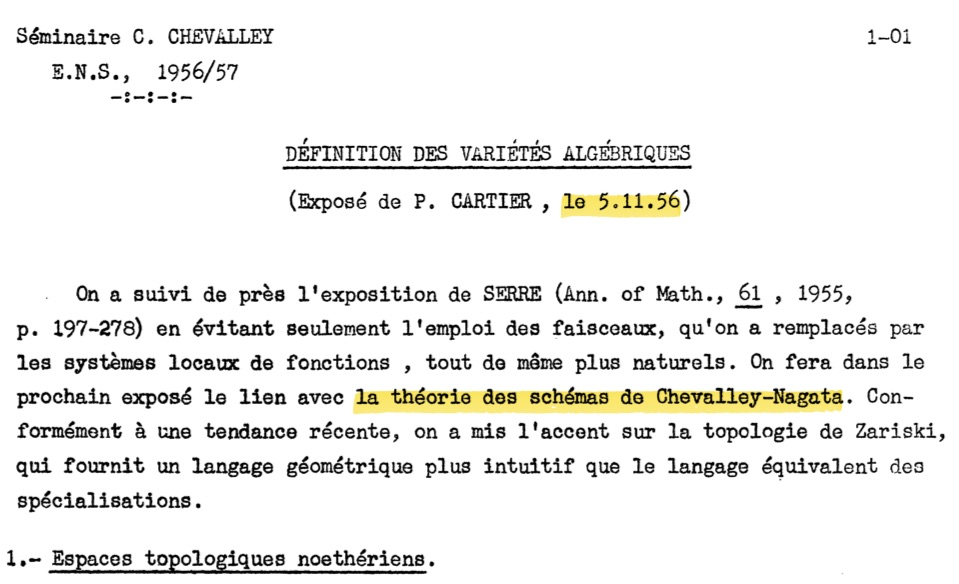In the 1956/57 Chevalley seminar Cartier gave the first two talks and in the first one, on november 5th 1956, one finds the first published use of the word ‘scheme’, which he refers to as ‘schemes in the sense of Chevalley-Nagata’. On page 9 of that talk he introduces the prime spectrum with its Zariski topology.

In the second talk a week later, on november 12th, he then gives the general definition of a scheme (as we know it, by gluing together affine schemes and including the stalks).

BUT, he did all of this ‘only’ for affine rings over a field, ‘to avoid an excessively long exposition of the preliminaries’…

Grothendieck then made the quantum-leap to general commutative rings.

June 18th, 2013

Correction : scheme-birthday = december 12th, 1955

Claude Chevalley gave already two talks on ‘Schemes’ in the Cartan-Chevalley seminar of 1955/56, the first one on december 12th 1955, the other a week later.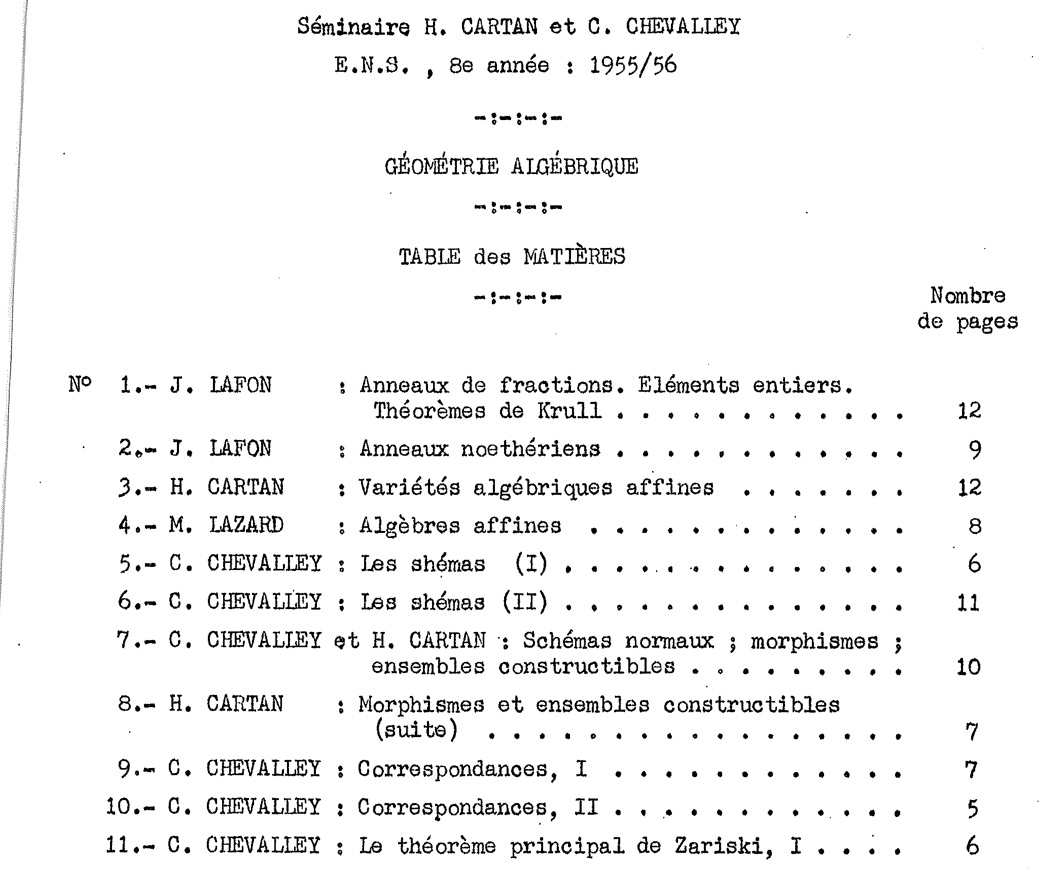Chevalley only considers integral schemes, of finite type over a field (Cartier drops the integrality condition on november 5th 1956, a bit later Grothendieck will drop all restrictions).

Grothendieck’s quote “But then, what are schemes?” uttered in a Parisian Cafe must date from that period. Possibly Cartier explained the concept to him. In a letter to Serre, dated december 15th 1955, Grothendieck is quite impressed with Cartier:

“Cartier seems to be an amazing person, especially his speed of understanding, and the incredible amount of things he reads and grasps; I really have the impression that in a few years he will be where you are now. I am exploiting him most profitably.”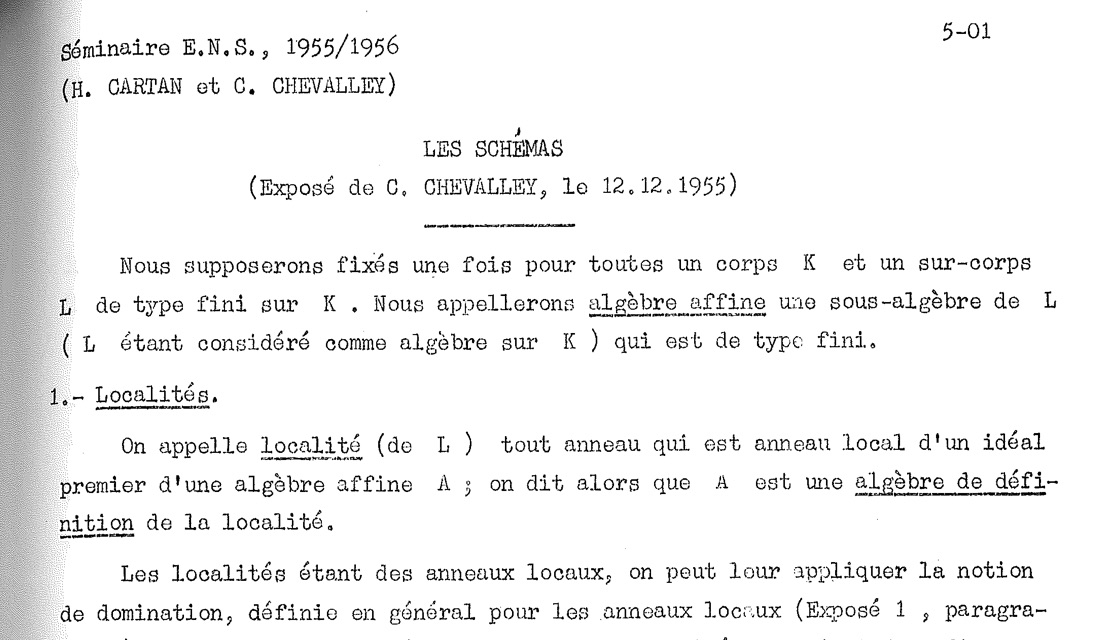June 18th, 2013

David Mumford on the Italian school of Geometry

Short version:
Castelnuovo : the good
Enriques : the ugly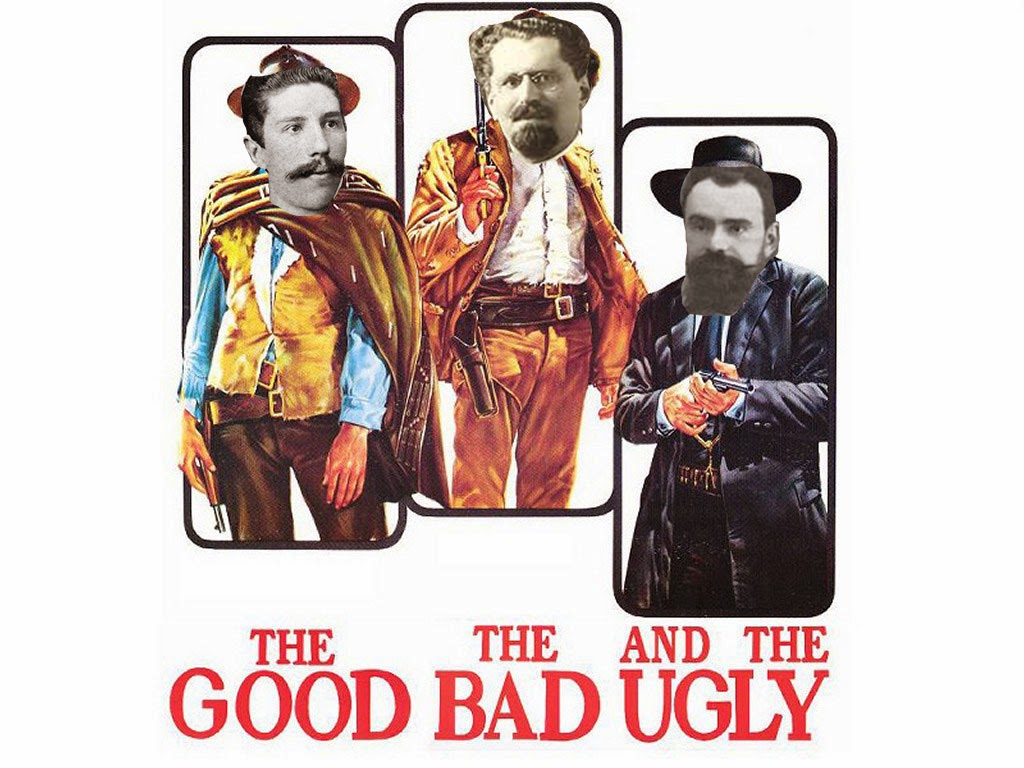The longer version:

“The best known case is the Italian school of algebraic geometry, which produced extremely good and deep results for some 50 years, but then went to pieces.
There are 3 key names here — Castelnuovo, Enriques and Severi.

C was earliest and was totally rigorous, a splendid mathematician.

E came next and, as far as I know, never published anything that was false, though he openly acknowledged that some of his proofs didn’t cover every possible case (there were often special highly singular cases which later turned out to be central to understanding a situation).  He used to talk about posing “critical doubts”. He had his own standards and was happy to reexamine a “proof” and make it more nearly complete.

Unfortunately Severi, the last in the line, a fascist with a dictatorial temperament, really killed the whole school because, although he started off with brilliant and correct discoveries, later published books full of garbage (this was in the 30’s and 40’s). The rest of the world was uncertain what had been proven and what not. He gave a keynote speech at the first Int Congress after the war in 1950, but his mistakes were becoming clearer and clearer.

It took the efforts of 2 great men, Zariski and Weil, to clean up the mess in the 40’s and 50’s although dredging this morass for its correct results continues occasionally to this day.” (David Mumford)

June 19th, 2014

Hirune Mendebaldeko – Bourbaki’s muse

After more than 70 years, credit is finally given to a fine, inspiring and courageous Basque algebraic geometer.

One of the better held secrets, known only to the first generation Bourbakistas, was released to the general public in april 2012 at the WAGS Spring 2012, the Western Algebraic Geometry Symposium, held at the University of Washington.

Hirune Mendebaldeko was a Basque pacifist, a contemporary of Nicholas Bourbaki, whom she met in Paris while there studying algebraic geometry. They were rumored to be carrying on a secret affair, with not infrequent trysts in the Pyrenees. Whenever they appeared together in public, however, there was no indication of any personal relationship.From the comments, by +Sandor Kovacs:

+Chris Brav Chris, just between us: the whole thing is a joke. I just tried to put yet another twist on it. Also, until now we have never admitted that it is, so please don’t tell anyone. 😉

The explanation at the end of +lieven lebruyn’s blog post was indeed the original motivation for the name. We were starting a new “named” lecture series as part of WAGS and wanted to name it after someone not obvious. Basque is a language not related to any other. It seemed a good idea to use that, so very few people would know the meaning of any particular word.

Then we tried all the words in WAGS, but the other three were actually very similar to the English/etc versions. The first name was chosen by vibe. Then we decided that we needed a bio for our distinguished namesake and the connection to Bourbaki presented itself for various reasons that you can guess. But we wanted a pacifist and it seemed a nice contrast to Bourbaki. So Hirune was born and we were hoping that one day she would gain prominence in the world. Finally, it happened. 🙂

June 22nd, 2014

the state of European mathematics in 1927

This map, from the Rockefeller foundation, gives us the top 3 mathematical institutes in 1927 : Goettingen, Paris and … Rome.
The pie-charts per university show that algebra was a marginal topic then (wondering how a similar map might look today).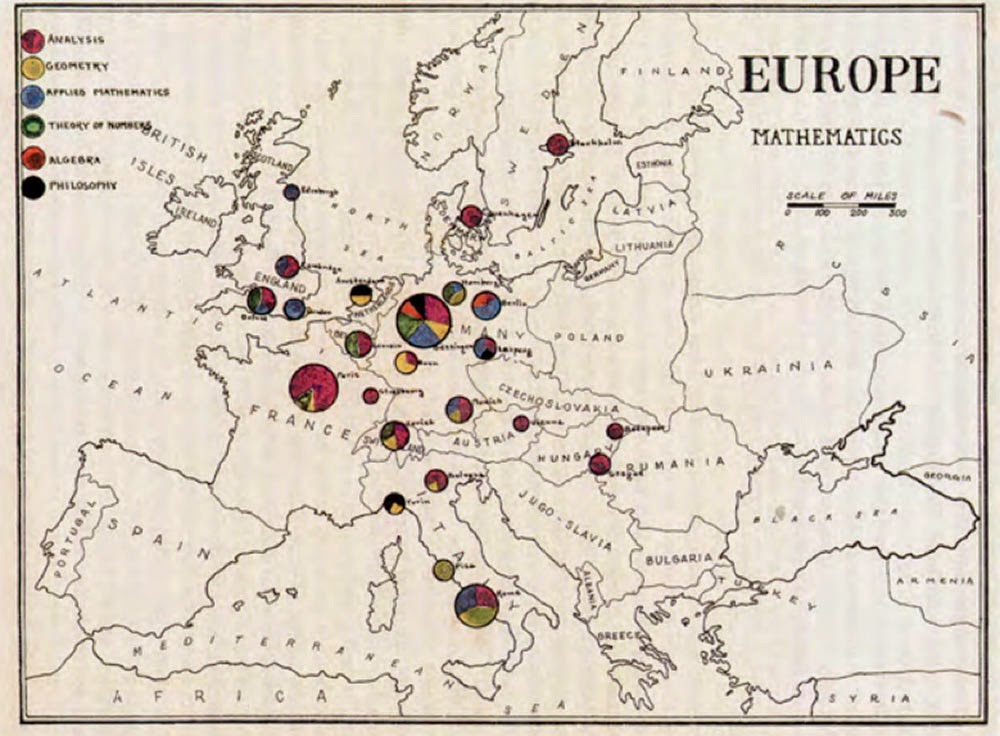December 1st, 2015

Did Chevalley invent the Zariski topology?

In his inaugural lecture at #ToposIHES Pierre Cartier stated (around 44m11s):
“By the way, Zariski topology, as we know it today, was not what Zariski invented. He invented a variant of that, a topology on the set of all valuation rings of a given field, which is not exactly the same thing. As for the Zariski topology, the rumour is that it was invented by Chevalley in a seminar given by Zariski, but I have no real proof.”

Btw. the full lecture of Cartier (mostly on sheaf theory) is not on the IHES YouTube channel, but on the channel of +Laurence Honnorat.

The IHES did begin to upload videos of the remaining plenary talks
here (so far, the wednesday talks are available).

The final part, starting with a reaction of Mochizuki himself on my previous IUTeich-posts.

July 7th, 2013

Mochizuki update

While i’ve been away from G+, someone emailed Mochizuki my last post on the problem i have with Frobenioids1. He was kind enough to forward  me M’s reply:

“There is absolutely nothing difficult or subtle going on here (e.g., by comparison to the portion of the theory cited in the discussion preceding the statement of this “problem”!).  The nontrivial result is the fact that the degree is rational, i.e., the initial portion of [FrdI], Theorem 6.4, (iii), which is a consequence of a highly nontrivial result in transcendence theory due to Lang (i.e., [FrdI], Lemma 6.5, (ii)).  Once one knows this rationality, the conclusion that distinct prime numbers are not confused with one another is a formal consequence (i.e., no complicated subtle arguments!) of the fact that the ratio of the natural logarithm of any two distinct prime numbers is never rational.
Sincerely, Shinichi Mochizuki”

Being back, i’ll give it another go.

Having read the shared article below (2/5/2019 ‘the paradox of the proof’), it’s comforting to know that other people, including  +Aise Johan de Jong and +Cathy O’Neil, are also frustrated by M’s opaque latest writings.

July 11th, 2013

Mochizuki’s Frobenioid reconstruction: the final bit

In “The geometry of Frobenioids 1” Mochizuki ‘dismantles’ arithmetic schemes and replaces them by huge categories called Frobenioids. Clearly, one then wants to reconstruct the schemes from these categories and we almost understood how he manages to to this, modulo the ‘problem’ that there might be auto-equivalences of $Frob(\mathbb{Z})$, the Frobenioid corresponding to $\mathbf{Spec}(\mathbb{Z})$ i.e.the collection of all prime numbers, reshuffling distinct prime numbers.

In previous posts i’ve simplified things a lot, leaving out the ‘Arakelov’ information contained in the infinite primes, and feared that this lost info might be crucial to understand the final bit. Mochizuki’s email also points in that direction.

Here’s what i hope to have learned this week:

the full Frobenioid $Frob(\mathbb{Z})$

objects of $Frob(\mathbb{Z})$ consist of pairs $(q,r)$ where $q$ is a strictly positive rational number and $r$ is a real number.

morphisms are of the form $f=(n,a,(z,s)) : (q,r) \rightarrow (q’,r’)$ (where $n$ and $z$ are strictly positive integers, $a$ a strictly positive rational number and $s$ a positive real number) subject to the relations that

$q^n.z = q’.a \quad \text{and} \quad n.r+s = r’+log(a)$

$n$ is called the Frobenious degree of $f$ and $(z,s)$ the divisor of $f$.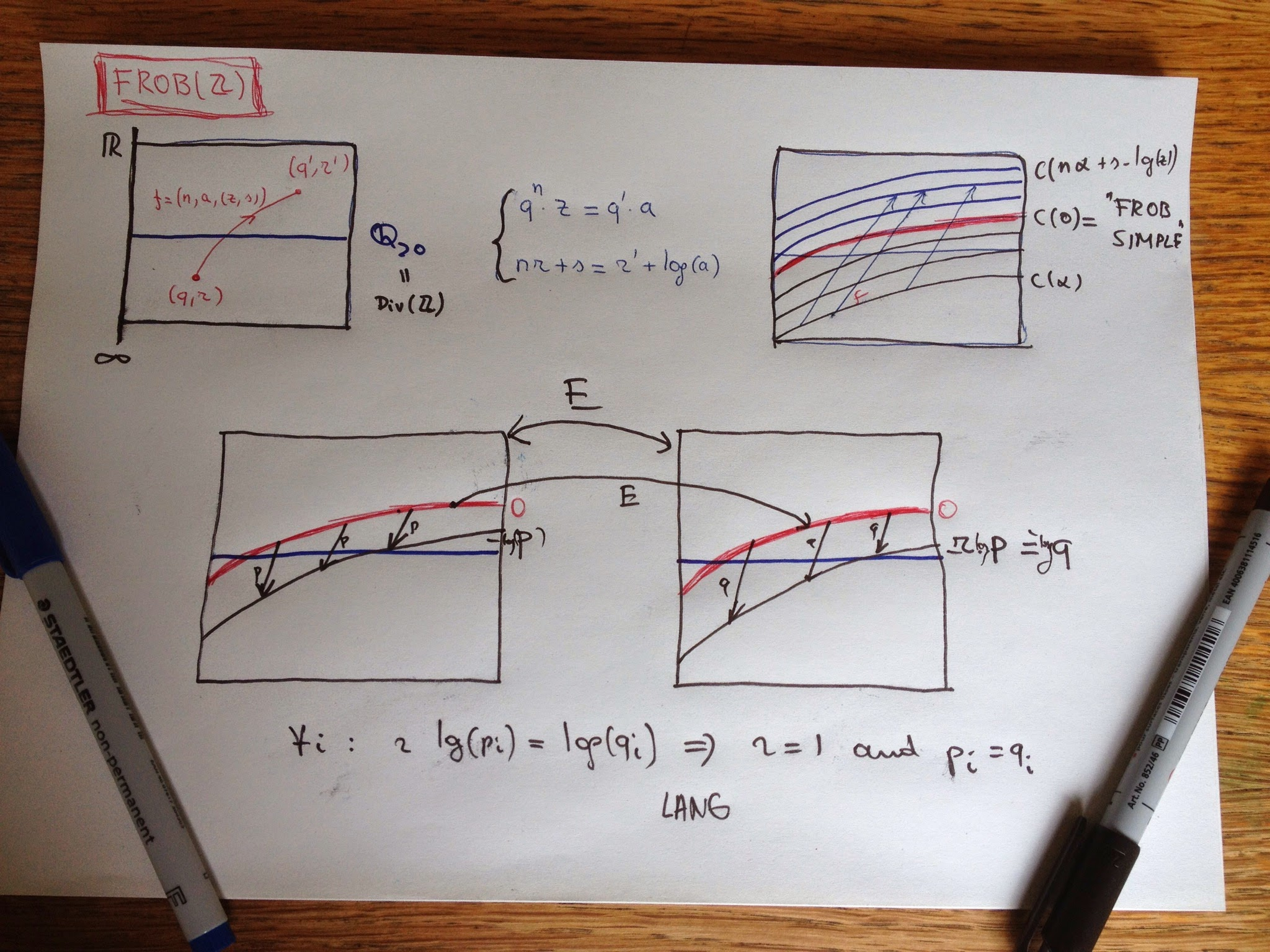All this may look horribly complicated until you realise that the isomorphism classes in $Frob(\mathbb{Z})$ are exactly the ‘curves’ $C(\alpha)$ consisting of all pairs $(q,r)$ such that $r-log(q)=\alpha$, and that morphisms with the same parameters as $f$ send points in $C(\alpha)$ to points in $C(\beta)$ where

$\beta = n.\alpha + s – log(a)$

So, the isomorphism classes can be identified with the real numbers $\mathbb{R}$ and special linear morphisms of type $(1,a,(1,s))$ and their compositions are compatible with the order-structure and addition on $\mathbb{R}$.

Crucial is Mochizuki’s observation that $C(0)$ are precisely the ‘Frobenious-trivial’ objects in $Frob(\mathbb{Z})$ (i’ll spare you the details but it is a property on having sufficiently many nice endomorphisms).

Now, consider an auto-equivalence $E$ of $Frob(\mathbb{Z})$. It will induce a map between the isoclasses $E : \mathbb{R} \rightarrow \mathbb{R}$ which is additive and as Frob-trivs are mapped under $E$ to Frob-trivs this will map $0$ to $0$, so $E$ will be an additive group-endomorphism on $\mathbb{R}$ hence of the form $x \rightarrow r.x$ for some fixed real number $r$, and we want to show that $r=1$.

Linear irreducible morphisms are of type $(1,a,(p,0))$ where $p$ is a prime number and they map $C(\alpha)$ to $C(\alpha-log(p))$. As irreducibles are preserved under equivalence this means that for each prime $p$ there must exist a prime $q$ such that $r log(p) = log(q)$.

Now if $r$ is an irrational number, there must be at least three triples $(p_1,q_1),(p_2,q_2)$ and $(p_3,q_3)$ satisfying $r log(p_i) = log(q_i)$ but this contradicts a fairly hard result, due to Lang, that for 6 distinct primes l_1,…,l_6 there do not exist positive rational numbers $a,b$ such that

$log(l_1)/log(l_2) = a log(l_3)/log(l_4) = b log(l_5)/log(l_6)$

So, $r$ must be rational and of the form $n/m$, but then $r=1$ (if not the correspondence $r.log(p) = log(q)$ gives $p^n=q^m$ contradicting unique factorisation). This then shows that under the auto-equivalence each prime $p$ (corresponding to a linear irreducible map) is send to itself.

This was the remaining bit left to show that the Frobenioid corresponding to any Galois extension of the rationals contains enough information to reconstruct from it the schemes of all rings of integers in intermediate fields.

August 15th, 2013

For travellers into Mochizuki-territory the indispensable rough guide is Lucien Szpiro’s ‘Marabout-Flash de théorie des nombres algébriques’, aka section I.1.3 in  ‘Séminaire sur les pinceaux arithmétiques: la conjecture de Mordell’.

Marabout Flash was a Franco-Belge series of do-it-yourself booklets, quite popular in the 60ties and 70ties, on almost every aspect of everyday’s life.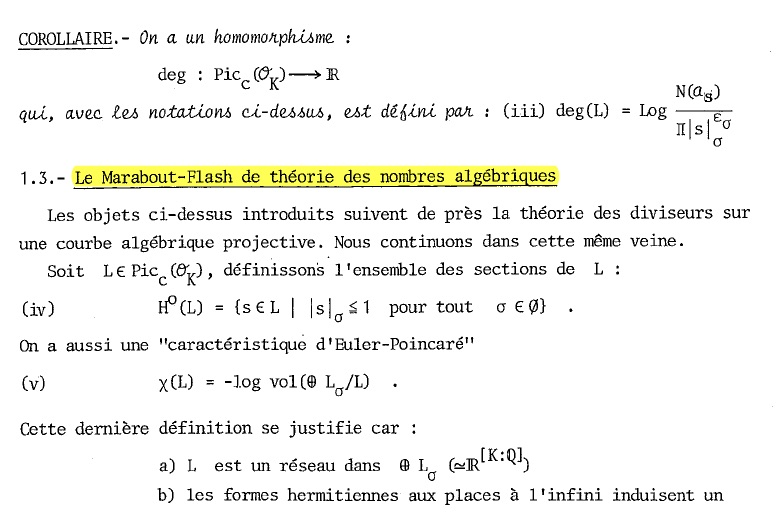In just a couple of pages Szpiro describes how one can extend the structure sheaf of a fractional ideal of a ring of integers to a ‘metrized’ (or Arakelov) line-bundle on the completed prime spectrum (including the infinite places). These bundles then satisfy properties similar to those of line-bundles on smooth projective curves, including a version of the Riemann-Roch theorem and a criterium to have non-zero global sections.

These (fairly simple) results then quickly lead to proofs of the first major results in number theory such as the Hermite-Minkowski theorem and Dirichlet’s unit theorem.

December 29th, 2014

Mochizuki in denial

From M’s 2014 IUTeich-Progress-Report (17 pages, the 2013-edition was only 7 pages long):

“Activities surrounding IUTeich appears to be in a stage of transition from a focus on verification to a focus on dissemination.”

If only…

He further lists hypotheses as to why nobody (apart from his 3 disciples Yamashita,Saidi and Hoshi) makes a serious effort to “study the theory carefully and systematically from the beginning”:

1. it is too long (1500-2500 pages)
2. there’s lack of textbooks on anabelian geometry
3. we are obsessed with the Langlands program
4. there’s little room for generalisations
5. it may not be directly useful for our own research

But then, why should anyone make such an effort, as:

“With the exception of the handful of researchers already involved in the verification activities concerning IUTeich, every researcher in arithmetic geometry throughout the world is a complete novice with respect to the mathematics surrounding IUTeich, and hence, in particular, is simply not qualified to issue a definite judgment concerning the validity of IUTeich on the basis of a ‘deep understanding’ arising from his/her previous research achievements.”

No Mochizuki, the next phase will not be dissemination, it will be denial.

Other remarkable sentences are:

“IUTeich is ‘the correct theory’ in the sense that it leads one to doubt the existence of any sort of ‘alternative proof’, i.e. via essentially different techniques, of the ABC Conjecture.”

and:

“the status of IUTeich in the field of arithmetic geometry constitutes a sort of faithful miniature model of the status of pure mathematics in human society.”

Already looking forward to the 2015 ‘progress’ report…

October 8th, 2015

Proud to be working at a well-known university

First time I’m mentioned in “Nature”, they issue this correction:

Corrected: An earlier version of this story incorrectly located the University of Antwerp in the Netherlands. It is in Belgium. The text has been updated.

Not particularly proud of the quote they took from my blog though:

“Is it just me, or is Mochizuki really sticking up his middle finger to the mathematical community”.

December 17th, 2017

Yesterday, my feeds became congested by (Japanese) news saying that Mochizuki’s (claimed) proof of the abc-conjecture had been vetted and considered fit to be published in a respectable journal.

Today, long term supporters of M’s case began their Echternachian-retreat after finding out that Mochizuki himself is the editor in charge of that respectable journal.

Attached is Ed Frenkel’s retraction of his previous tweet. Also, Taylor Dupuy deemed the latest action a bridge too far, see here.

Peter Woit did a great job in his recent post
pinpointing a critical argument in the 500-page long papers having as its “proof” that it followed trivially from the definitions…

I’d expect any board of editors to resign in such a case.
I fear this can only end badly.

Here’s batch 2 of my old google+ posts on ‘Inter Universal Teichmuller theory’, or rather on the number theoretic examples of Frobenioids.

June 5th, 2013

Mochizuki’s categorical prime number sieve

And now for the interesting part of Frobenioids1: after replacing a bunch of arithmetic schemes and maps between them by a huge category, we will reconstruct this classical picture by purely categorical means.

Let’s start with the simplest case, that of the ‘baby arithmetic Frobenioid’ dismantling $\mathbf{Spec}(\mathbb{Z}) (that is, the collection of all prime numbers) and replacing it by the category having as its objects all$(a)$where$a$is a strictly positive rational number and morphisms labeled by triples$(n,r,z)$where$n$and$z$are strictly positive integers and$r$is a strictly positive rational number and connecting two objects $(n,r,z) : (a) \rightarrow (b) \quad \text{ if and only if } \quad a^n.z=b.r$ Composition of morphisms is well-defined and looks like$(m,s,v) \circ (n,r,u) = (m.n,r^m.s,u^m.v)$as one quickly checks. The challenge is to recover all prime numbers back from this ‘Frobenioid’. We would like to take an object$(a)$and consider the maps$(1,1,p)$from it for all prime numbers$p$, but cannot do this as categorically we have to drop all labels of objects and arrows. That is, we have to recognize the map$(1,1,p)$among all maps starting from a given object.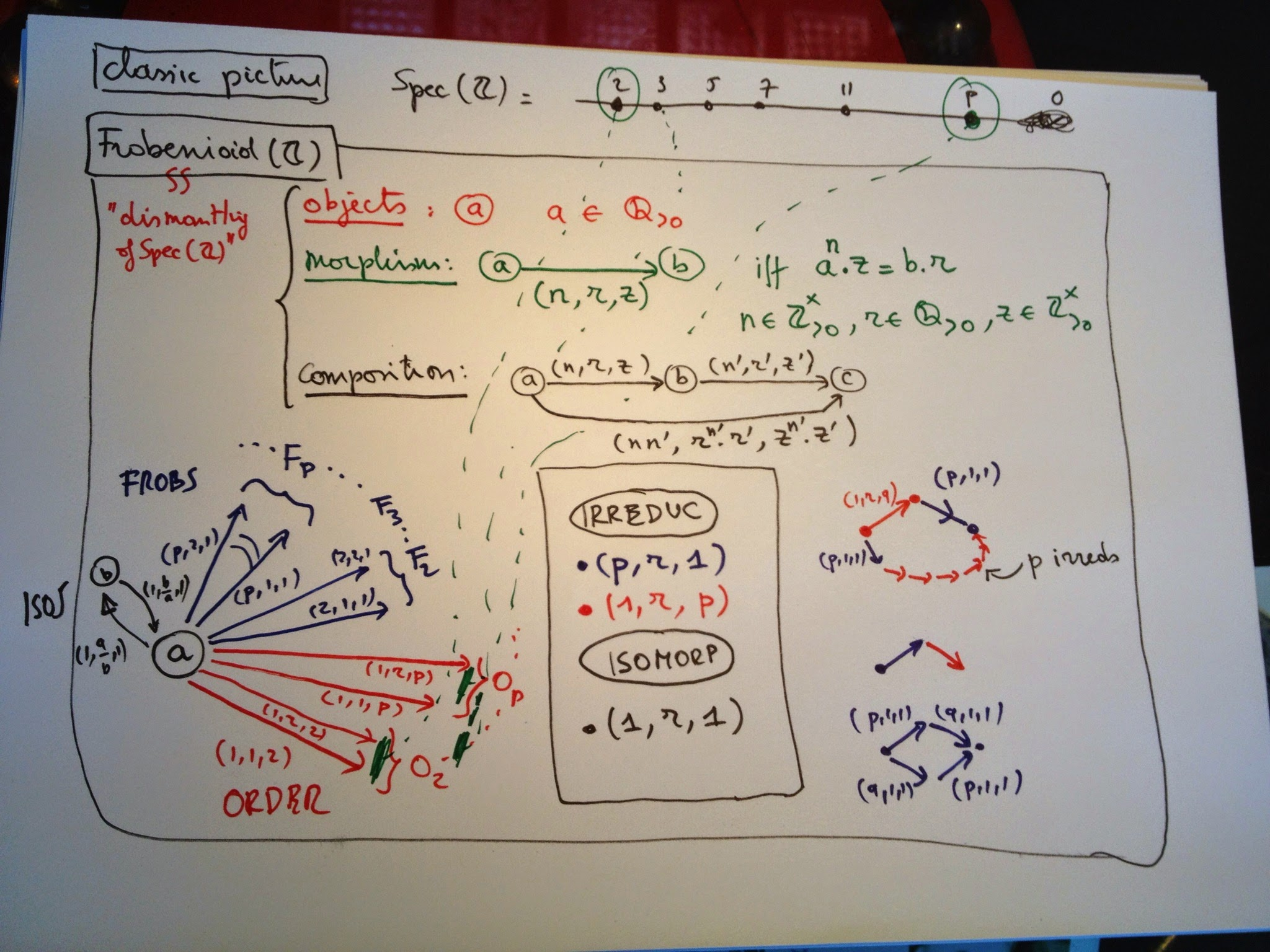We can identify all isomorphisms in the category and check that they are precisely the morphisms labeled$(1,r,1)$. In particular, this implies that all objects are isomorphic and that there is a natural correspondence between arrows leaving$(a)$and arrows leaving$(b)$by composing them with the iso$(1,b/a,1) : (b) \rightarrow (a)$. Another class of arrows we can spot categorically are the ‘irreducibles’, which are maps$f$which are not isos but have the property that in any factorization$f=g \circ h$either$g$or$h$must be an iso. One easily verifies from the composition rule that these come in two flavours: – those of Frobenius type :$(p,r,1)$for any prime number$p$– those of Order type :$(1,r,p)$for any prime number$p$We would like to color the froBs Blue and the oRders Red, but there seems to be no way to differentiate between the two classes by purely categorical means, until you spot Mochizuki’s clever little trick. start with a Red say$(1,r,q)$for a prime number$q$and compose it with the Blue$(p,1,1)$, then you get the morphism$(p,r^p,q^p)$which you can factor as a composition of$p+1$irreducibles $(p,r^p,q^p) = (1,r,q) \circ (1,r,q) \circ …. \circ (1,r,q)o(p,1,1)$ and if$p$grows, so will the number of factors in this composition. On the other hand, if you start with a Blue and compose it with either a Red or a Blue irreducible, the obtained map cannot be factored in more irreducibles. Thus, we can identify the Order-type morphisms as those irreducibles$f$for which there exists an irreducible$g$such that the composition$g \circ f$can be factored as the composition in at least$n$irreducibles, where we can take n arbitrarily large. Finally we say that two Reds out of$(a)$are equivalent iff one is obtained from the other by composing with an isomorphism and it is clear that the equivalence classes are exactly the arrows labeled$(1,r,p)$for fixed prime number$p$. So we do indeed recover all prime numbers from the category. Similarly, we can see that equivalence classes of Frobs from$(a)$are of the form$(p,r,1)$for fixed prime$p$. An amusing fact is that we can recover the prime$p$for a Frob by purely categorical ways using the above long factorization of a composition with a Red. There seems to be no categorical way to determine the prime number associated to an equivalence class of Order-morphisms though… Or, am i missing something trivial? June 7th, 2013 Mochizuki’s Frobenioids for the Working Category Theorist Many of you, including +David Roberts +Charles Wells +John Baez (and possibly others, i didn’t look at all comments left on all reshares of the past 4 posts in this MinuteMochizuki project) hoped that there might be a more elegant category theoretic description of Frobenioids, the buzz-word apparently being ‘Grothendieck fibration’ … Hence this attempt to deconstruct Frobenioids. Two caveats though: – i am not a category theorist (the few who know me IRL are by now ROFL) – these categories are meant to include all arithmetic information of number fields, which is a messy business, so one should only expect clear cut fibrations in easy situation such as principal ideal domains (think of the integers$\mathbb{Z}$). Okay, we will try to construct the Frobenioid associated to a number field$K$(that is, a finite dimensional extension of the rationals$\mathbb{Q}$) with ring of integers$R$(the integral closure of$\mathbb{Z}$in$K$). For a concrete situation, look at the quadratic case. The objects will be fractional ideals of K which are just the R-submodules$I$of$K$such that there in an$r$in$R$such that$I.r$is a proper ideal of$R$. Dedekind showed that any such thing can be written uniquely as a product $I = P_1^{a_1} … P_k^{a_k}$ where the$P_i$are prime ideals of$R$and the$a_i$are integers (if they are all natural numbers, I will be a proper ideal of$R$). Clearly, if one multiplies two fractional ideals$I$and$J$, the result$I.J$is again a fractional ideal, so they form a group and by Dedekind’s trick this group is the free Abelian group on all prime ideals of$R$.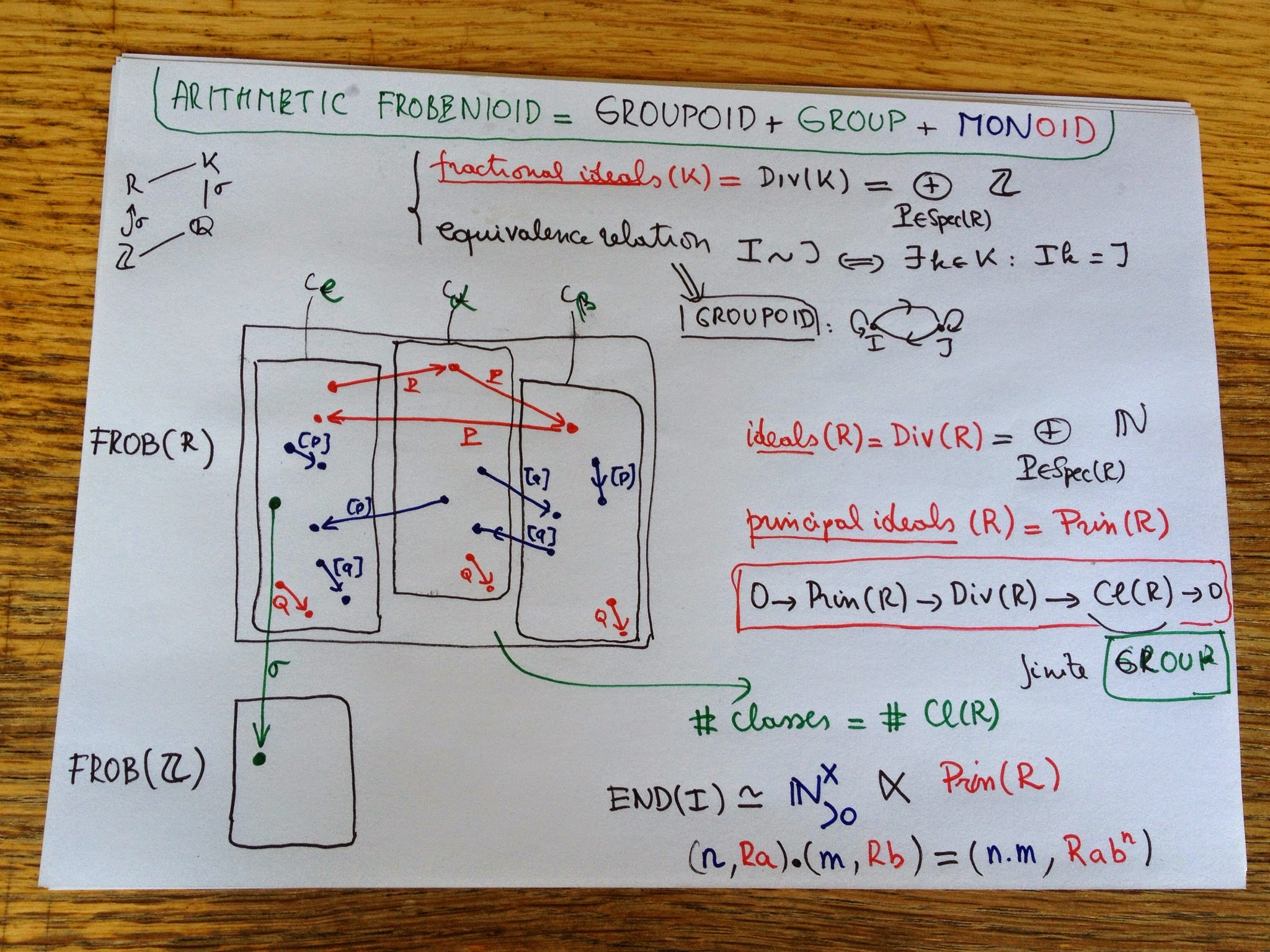Next, we define an equivalence relation on this set, calling two fractional ideals$I$and$J$equivalent if there is a$k$in$K$such that$I=J.k$(or if you prefer, if they are isomorphic as$R$-modules). We have a set with an equivalence relation and hence a groupoid where these is a unique isomorphism between any two equivalent objects. This groupoid is precisely the groupoid of isomorphisms of the Frobenioid we’re after. The number of equivalence classes is finite and these classes correspond to the element of a finite group$Cl(R)$called the class group of$R$which is the quotient group of ideals modulo principal ideals (so if your$R$is a principal ideal domain there is just one component). The ‘groups’ corresponding to each connected component of the groupoid are all isomorphic to the quotient group of the units in$K$by the units in$R$. Next, we will add the other morphisms. By definition they are all compositions of irreducibles which come in 2 flavours: – the order-morphisms$P$for any prime ideal$P$of$R$sending$I$to$I.P$. Typically, these maps switch between different equivalence classes (unless$P$itself is principal). We can even explicitly compute small norm prime ideals which will generate all elements in the class group$Cl(R)$. – the power-maps$[p]$for any prime number$p$which sends$I$to$I^p$. The nature of these maps really depend on the order of the component in the finite group$Cl(R)$. Well, that’s it basically for the layer of the Frobenioid corresponding to the number field$K$. (You have to repeat all this for any subfield between$\mathbb{Q}$and$K$). A cute fact is that all endomorphism-monoids of objects in the layer of K are all isomorphic as abstract monoid to the skew-monoid$\mathbb{N}^x_{>0} x Prin(R)$of the multiplicative group of all strictly positive integers$n$with the monoid of all principal ideals in R with multiplication defined by $(n,Ra).(m,Rb)=(nm,Rab^n)$ The only extra-type morphisms we still have to include are those between the different layers of the Frobenioid, the green ones which M calls the pull-back morphisms. They are of the following form: if$R_1$and$R_2$are rings of integers in the fields$K_1$contained in$K_2$, then for any ringmorphism$\sigma : R_1 \rightarrow R_2$one can extend a fractional ideal$I$of$R_1$to$K_2$by considering$R_2.\sigma(I)$. These then give the morphism$r_2\sigma(I) \rightarrow I$and as we will see in a next instalment, they encode the splitting behaviour of prime ideals. a question for category people Take the simplest situation, that of the integers$\mathbb{Z}$. So, we have just a groupoid with extra morphisms generated by the order-maps$o_p$and the power maps$f_p$. The endo-ring of any object is then isomorphic top the abstract group generated by the$f_p$and$o_p$and satisfying following relations $o_p.o_q=o_q.o_p$ $f_p.f_q=f_q.f_p$ $f_p.o_q=o_q^p.f_p$ My question now is: if for two different primes$p$and$q$i switch their role in the endo-ring of 1 object and propagate this via all isos to all morphisms, do i get a category equivalence? (or am i missing something?). (tbc) June 11th, 2013 my problem with Mochizuki’s Frobenioid1 Let us see how much arithmetic information can be reconstructed from an arithmetic Frobenioids. Recall that for a fixed finite Galois extension$L$of$\mathbb{Q}$this is a category with objects all fractional ideals in subfields of$L$, and maps generated by multiplication-maps with ideals in rings of integers, power-maps and Galois-extension maps. When all objects and morphisms are labeled it is quite easy to reconstruct the Galois field$L$from it as well as all maps between prime spectra of rings of integers in intermediate fields, which after all was the intended use of Frobenioids, to ‘dismantle’ these arithmetic schemes and endow them with extra structure given by the power-maps.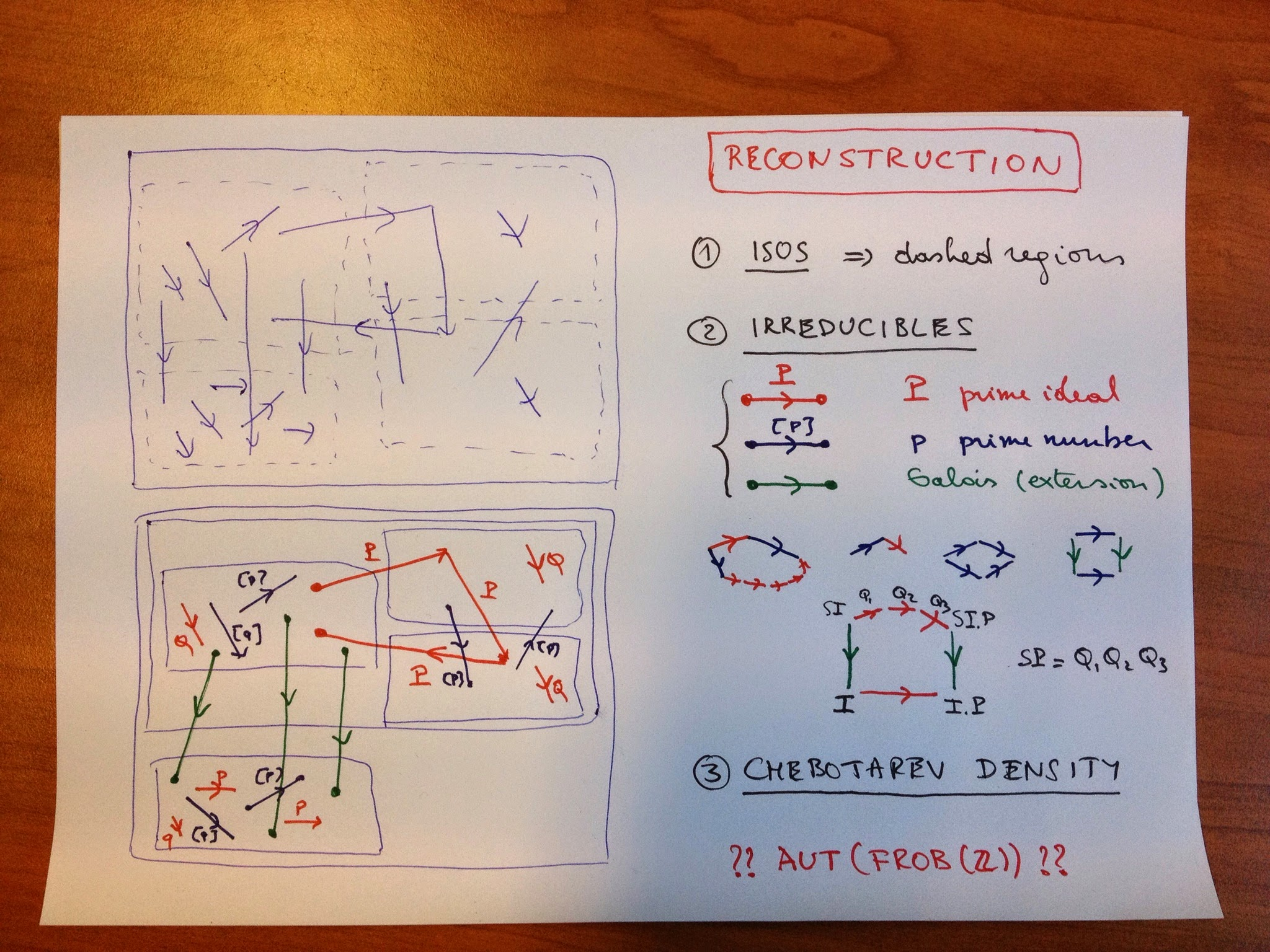However, in this reconstruction process we are only allowed t use the category structure, so all objects and morphisms are unlabelled (the situation top left) and we want to reconstruct from it the different layers of the Frobenioid (corresponding to the different subfields) and divide all arrows according to their type (situation bottom left). First we can look at all isomorphisms. They will divide the category in the dashed regions, some of them will be an entire layer (for example for$\mathbb{Q}$) but in general a finite number of these regions will make up the full layer of a subfield (the regions labeled by the elements of the ideal class group). Another categorical notion we can use are ‘irreducible morphisms’, that is a morphism$f$which is not an iso but having the property that in each factorisation$f = g \circ h$either$g$or$h$must be an iso. If we remember the different types of morphisms in our Frobenioid we see that the irreducibles come in 3 flavours: – oRder-maps (Red) : multiplication by a prime ideal$P$of the ring of integers of the subfield – froBenius or power-maps (Blue) sendingg a fractional ideal$I$to$I^p$for a prime number$p$– Galois-maps (Green) extending ideals for a subfield$K$to$K’$having no intermediate field. We would like to determine the colour of these irreducibles purely categorical. The idea is that reds have the property that they can be composed with another irreducible (in fact, of power type) such that the composition can again be decomposed in irreducibles and that there is no a priori bound on the number of these terms (this uses the fact that$[p] \circ Q = Q \circ Q \circ … Q \circ [p]$and that there are infinitely many prime numbers$p$). One checks that compositions of order or Galois maps with irreducibles have factorisation with a bounded number of irreducibles. The most interesting case is the composition of a Galois map with an order map$P$, this can be decomposed alternatively as order maps in the bigger field followed by a Galois map, the required order maps are the bigger primes$Q_i$occurring in the decomposition of the extended ideal $S.P = Q_1.Q_2….Q_k$ but the number$k$of this decomposition is bounded by the dimension of the bigger field over the smaller one. Summarizing: – we can determine all the red maps, which will then give us also the different layers – we can determine the green maps as they move between different layers – to the remaining blue ones we can even associate their label$[p]$by the observed property of composition with order maps. Taking an object in a layer, we get the set of prime ideals of the ring on integers in that field as the set of all red arrows leaving that object unto equivalence (by composing with an isomorphism), so we get the prime spectra$\mathbf{Spec}(S)$. For a ring-extension$R \rightarrow S$we also can recover the cover map$\mathbf{Spec}(S) \rightarrow \mathbf{Spec}(R)$Indeed, composing the composition of the Galois map with the order-map$P$and decomposing it alternatively will give us the finite number of prime ideals$Q_i$of$S$lying over$P$. That is, we get all splitting behaviour of prime ideals in intermediate field-extensions. Let$K$be a Galois subfield of$L$then we have a way to see how a prime ideals in$\mathbf{Spec}(\mathbb{Z})$splits, ramifies or remains inert in$K$and so by Chebotarev density this gives us the dimension of$K$as well as the Galois group. And, if we could label the prime ideal by a prime number$p$, we could even reconstruct$K$itself as$K$is determined once we know all prime numbers which completely split. The problem i have is that i do not see a categorical way to label the red arrows in$Frob(\mathbb{Z})$by prime numbers. Mochizuki says we can do this in the proof of Thm 6.4(iii) by using the fact that the$log(p)$are linearly independent over$\mathbb{Q}$. This suggests that one might use the ‘Arakelov information’ (that is the archimidean valuations) to do this (the bit i left out so far), but i do not see this in the case of$\mathbb{Q}\$ as there is just 1 extra (real) valuation determined by the values of the nonarchimidean valuations.

Probably i am missing something so all sorts of enlightenment re welcome!